# Exploring fastai with Excel and Python

This topic is a test on how far I can explore theories and techniques taught in fastai using Excel with great help and guidance from @Moody and many others in fastai community.

Why Doing it?

• Jeremy said it multiple times Excel is greatly underappreciated by many in deep learning
• On how to do fastai, Jeremy said numerous times: just finish one thing and if you can, finish it nicely!
• I am deeply inspired by @Moody’s story with excel and fastai
• I want exploring fastai with detailed note and excel experiments to be my thing.
• I want to truly test my tenacity this time. (I was ashamed when Jeremy and Radek said you can only fail fastai by giving up. Just imagine it, I joined the forum at the end of 2016. Pray for no accidents this time)

What is the plan

5 Likes

## Excel tips

• How to draw graph using columns of data?

• How to reuse Jeremy’s macros for all my workbooks?

• open project explorer
• open Jeremy’s excel file and your workbook
• copy and paste the macro to the worksheet you want it to have
• How to debug or inspect data flow in your formula?

• How to write a custom VBA function with docs?

• How to construct a complex Lambda + Let function step by step in Excel?

## Experiment and contemplation

Questions experimented

# Notes from fastai teaching

You can search all fastai videos with keywords! Thank you very much @fmussari for this wonderful tool!

#### What does Jeremy say about epochs?

Summary
• What is an epoch?
• What could happen if you run 10 epochs with lots of weights and high learning rate?
• What would happen related to overfitting if you do just 1 epoch?

Are these Jeremy’s experimental knowledge or there is some more blogs or papers can read upon?

#### Do weight_decay vs l2_reg behave differently under the same but more complex optimization stepping functions?

2 Likes

Cautions: The tips I provided here are just to get you started. They are not the best practice to use Excel in terms of optimizing performance and memories. But, it seems you have so much fun already.2 Likes

How should we think of deep learning jargon according to Jeremy?

1 Like

## Why non-linear function is the power source of neuralnet from a mathematical perspective?

Quote from the fastbook

Amazingly enough, it can be mathematically proven that this little function can solve any computable problem to an arbitrarily high level of accuracy, if you can find the right parameters for `w1` and `w2` and if you make these matrices big enough. For any arbitrarily wiggly function, we can approximate it as a bunch of lines joined together; to make it closer to the wiggly function, we just have to use shorter lines. This is known as the universal approximation theorem . The three lines of code that we have here are known as layers . The first and third are known as linear layers , and the second line of code is known variously as a nonlinearity , or activation function .

1 Like

I was playing with the Titanic spreadsheet from the course repository , watching again Lesson 3 and was wondering how to replicate the linear regression in Google Sheets.

I didn’t knew of Google Sheets having a Solver (it seems that there are some implementations), but I explored other alternatives.

The first one I found was LINEST.

• LINEST is a function (also available in Excel) that “calculates various parameters about the ideal linear trend using the least-squares method”. When applying it, a loss of 0.396 was obtained.

So, according to the Google documentation it should do the job, but the Excel solver was giving us a much better result with loss of 0.1443.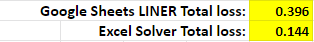In order to achieve a better result, I was wondering on how to apply Gradient Descent but wasn’t sure how to do it. I thought about Apps Script but was a bit reluctant to do it that way (I didn’t want slow for loops). I knew about this article of how to use Tensorflow.js inside Google Sheets: Machine Learning in Google Sheet with Tensorflow.js and Apps Script. I thought that maybe using ts.js loss functions or matrix operation would help me accomplish the objective, but not being proficient neither in Javascript or in Tensorflow would play against the objective.

But in the process I finally found all the Google Sheets formulas that allows to apply the Gradient Descent (in a relative simple way) and to get a result as good as Excel Solver.

#### Explore the file here: Titanic - Gradient Descent.

LINEST: The first parameters were obtained with the LINEST formula. As I mentioned, its results produced a loss of 0.396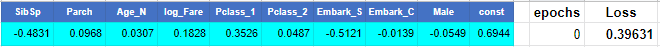The other functions used to calculate the gradients are the following:

SUMXMY2: Calculates the sum of the squares of differences of values between two arrays.
MMULT: Calculates the matrix product of two matrices specified as arrays or ranges.
TRANSPOSE: Transposes the rows and columns of an array or range of cells.

For calculating the partial derivatives a `delta` of 1E-6 was established.
So, the partials were calculated as follow:

``````∂v1 = (LOSS(v1, v2, ..., v10) - LOSS(v1+delta, v2, ..., v10)) / delta
∂v2 = (LOSS(v1, v2, ..., v10) - LOSS(v1, v2+delta, ..., v10)) / delta
(...)
∂v10 = (LOSS(v1, v2, ..., v10) - LOSS(v1, v2, ..., v10+delta)) / delta

``````

After doing 422 iterations and trying different Learning Rates, it was possible to get the same loss that was obtained with the Excel solver.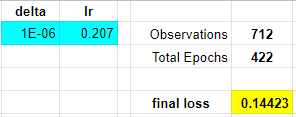#### Explore the file here: Titanic - Gradient Descent.

• I encourage you to play with diferente learning rate. The results are very susceptible to learning rate values.

To add an iteration, simply copy and paste lines from column M to AI.

3 Likes

Wow, thanks a lot for sharing! This is great!

I wonder when there will be fastai working with ExcelI will play with your googlesheet and get back to you later.

3 posts were merged into an existing topic: Python help

I also tried some fancy stuff like ipysheet (an ipywidget) in order to bring spreadsheet into Jupyter notebook. However, after two days of experiments I felt the widget is not robust enough to perform even though the design seem have all functionalities available.

I practiced Lesson 3 by implementing its Excel and Python parts in Google Sheets, I’m documenting here the experiments in case anyone is interested in tweaking some parameters and learning rates and see some plots reflecting those tweaks.

It is a also a resource in case anyone is interested in applying gradient descent with Google Sheets functions and try to fit a linear regression, a quadratic or the sum of 2 Rectified Linear Units (ReLU).

I agree that it is probably more useful to replicate these techniques with Python. The functions in Google Sheets may be getting a little big in order to achieve one iteration in one line. The reality is that the large functions (for loss and partial derivatives) are not written in one shot, they take multiple steps that are then grouped to avoid intermediate references. So they seem more complex that they are to create.

Anyway, the reason to publish this file and this post is based on the probability that they may become useful for someone at some point.

### Sheets in the Google Sheets file:

In the “Quadratic Manual” sheet you can manually change the green values for a, b, c. We need to change them to minimize the Loss. We can also see the plot of how the predictions get close to the points generated (those points are created by a base function and some noise added).

You can also change the base function (in this case 3x²+2x+1) by changing the blue a, b, c values.

This sheet applies Gradient Descend to automatically find the values a, b, c that best fit the data points (base function + noise).

One plot shows how the parameters a, b and c change in each iteration.
The other has the data points, the first prediction (quadratic with first approximation to parameters) and the quadratic with the final iteration (blue squares predictions).

It is interesting to see the difference by changing the learning rate from 0.004

to 0.005

With a learning rate of 0.006 the model doesn’t converge to find the parameters.

We can see how a big learning rate makes the big jumps of parameters a and c, between the values that produces the minimum loss, without the precision to reach that minimum. So, if we delete the last iteration, we can see how the predictions are jumping between the result.

To add or delete iterations, use copy and paste between columns G and N. All the other parameters and plot are generated automatically. Each row has the functions that calculate the loss and derivatives. Pasting one row should made each parameter approximate to the ones that minimizes the loss.
(Except for the case with a learning rate too big, look at the derivatives jumping from about -500 to +500 for parameter a and similar for parameter c).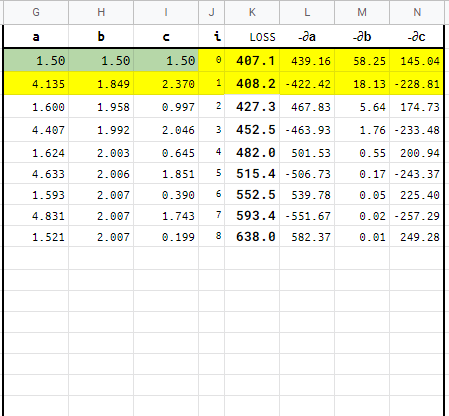In this sheet you can try to find the best m1, b1, m2, b2 in order to make 2 ReLU fit the same (or other) quadratic equation.

Gradient descent is again applied to find the best m1, b1, m2, b2.
Begining with base parameters as -2.00, 2.00, 2.00, 1.00. And a learning rate of 0.015.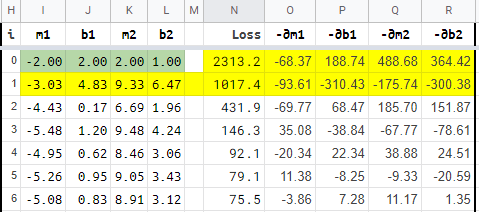After 36 iterations (copying and pasting), we end up with a loss of 69.26 (For the points generated with noise, the fundamental 3x²+2x+1 has a loss of 55.48).

With this model iterations are very sensitive to the changes in the learning rate or in the first values we choose. For example, if we change the initial m1 from -2.00 to 1.00 we get a not so good result: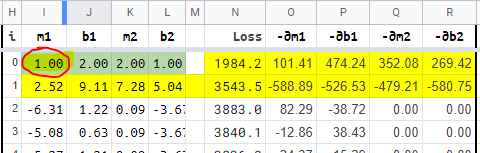The model stabilizes as a full straight line with a loss of 762.62.

But if we now change the learning rate from 0.015 to 0.010, the parameters undulates a little, but then converge to a loss of 66.29.

And you can start trying learning rates of 0.01, 0.012, 0.013 and find the minimum loss (with all else equal).

You can also try more iterations by copy and pasting butween H and R columns.

## Titanic 2ReLU [iter]

Here are two linear regressions, each with its own set of m1, m2, …, m9 and b1 parameters. Each iteration requires Google Sheets to calculate 20 derivatives. So changing some parameter or learning rate would need 20 derivatives for each iteration in the sheet. It takes about 1 minute for the 250 iterations that are defined in the sheet to update.

The model results and convergence gets very sensitive to the 20 different initial parameters an learning rates.

### First try

With the following initial parameters:

m11 m12 m13 m14 m15 m16 m17 m18 m19 b1 m21 m22 m23 m24 m25 m26 m27 m28 m29 b2
-0.48 0.10 0.03 0.18 0.35 0.05 -0.51 -0.01 -0.05 0.69 0.00 -0.10 -0.10 0.20 0.40 -0.50 1.00 0.00 -1.00 0.00

And a “big” learning rate of 0.35, we can see the process gets unstable after iteration 50.

### Second try

By only changing the learning rate to 0.19, the model converge and the “distribution” of final predictions looks more like the target distribution than the initial predictions:

## Titanic 2ReLU [iter] Solver

The initial aproximations used in this sheet are copied from predictions made with Excel Solver. So here the model is starting with very good parameters. So the iterations are to fine tune the already good parameters.

But what if we start the tuning with a leraning rate of 0.19?

### First try

As we can see, a very musical model, but not so good in converging.

### Second try

With a learning rate of 0.11 and 250 iterations, we get a small reduction in the loss from 0.13530 to 0.13363.

The two parameters that changed the most in the 250 iterations were m12 and m22…

## Conclusions

• Maybe not the best way to practice the concepts involved, but hopefully useful in some other ways.
• Would be fun to try some of the Sheets ideas or plots in python.
• Making this Sheet definitively gave me a good insight about gradient descent and ReLU.
• Tweaking the parameters in Sheets was a great way to see how changing a hyperparameter like the learning rate or the initial estimations of a parameter can affect the fitting process and its convergence.
4 Likes

# Questions on fastai docs

Hi @Moody, I have a question when reading and experimenting the source and doc of `Optimizer.__init__`

1. Does input `params` from `Optimizer.__init__` really contain hyper parameters?
2. What does `hyper parameters` mean here?
3. Don’t `**defaults` and `cbs``defaults` provide hyper parameters and their values?
4. According to the source code, `params` does not have to be a `Tensor`, nor `cbs` has to be a `list`, specifying their types to be `Tensor` and `list` is confusing.
5. `**defaults`: as `**` means keyword arguments, so it must be type `dict`. Can nbdev tell it automatically?

2 Likes

I have been silently picking a few things shared here in my day job too. Like to create small visualization with charts, using the formula’s in excel etc.

Thanks for creating this. I didn’t realize excel was this much powerful.

3 Likes

It may be easier for you to read the source code instead.

Per fastbook, `hyperparameters ... are parameters about parameters, since they are the higher-level choices that govern the meaning of the weight parameters.` (page 49 if you have a hard copy)

`defaults` are defined. With `**` in front of `defaults` is to unpack the contains.

Read the Optimizer code again in the repo and let us know when you learn.Python tips: Enter `?Optimizer` or `??Optimizer` in Jupyter. It will bring up the code as well. Click the `[Documentation]` on the top right-hand corner. It will take you to the doc.

1 Like

Could you have a look at this page of my nbdev project? I have found two tiny issues with `fastcore.delegates` and provided two simple solutions. I wonder what you think of them. Thank you!